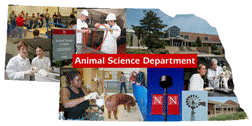## Animal Science Department## Faculty Papers and Publications in Animal Science

#### Date of this Version

March 1993

Published in J. Anim. Sci. 1993. 71:2006-2011.

#### Abstract

Henderson described a method to reduce the number of mixed-model equations when estimating additive and nonadditive genetic variances or predicting additive and nonadditive genetic merits. The extension to a maternal effects model is straightforward. When maternal genetic effects are strictly additive, an algebraic identity was found that reduces by a factor of two the order of a matrix that must be inverted each round to account for the genetic covariances among direct and maternal genetic effects. An algorithm for derivative-free restricted maximum likelihood was developed based on Henderson's total-merit model that is the basis for a reduced number of equations. The same values for the logarithm of the likelihood can be calculated from components of the equations for the total-merit model and from components of the equations for the individual effects model. The computational properties of the equations for the total-merit model, however, do not lend themselves to sparse-matrix methods. Both memory and time requirements were much greater for the total-merit model than for the individual-effects model for a data set of 871 animals and a model with additive, dominance, and additive x additive direct and additive maternal genetic effects. Approximately 14 times more memory was required, although the number of equations decreased from 3,773 to 2,031. Computing time per round increased by a factor of 50.

COinS